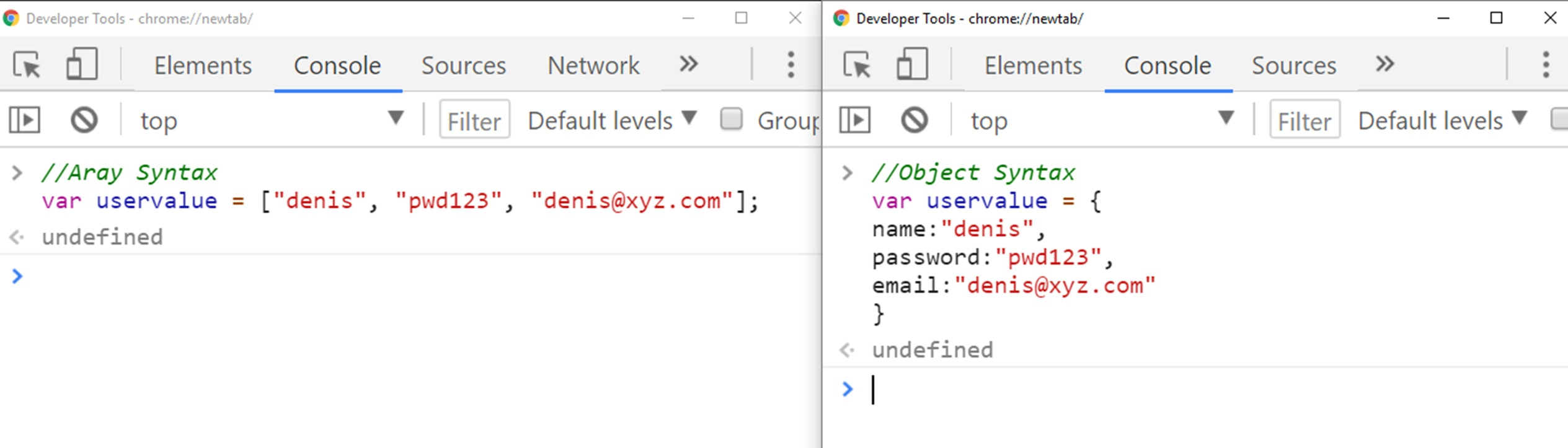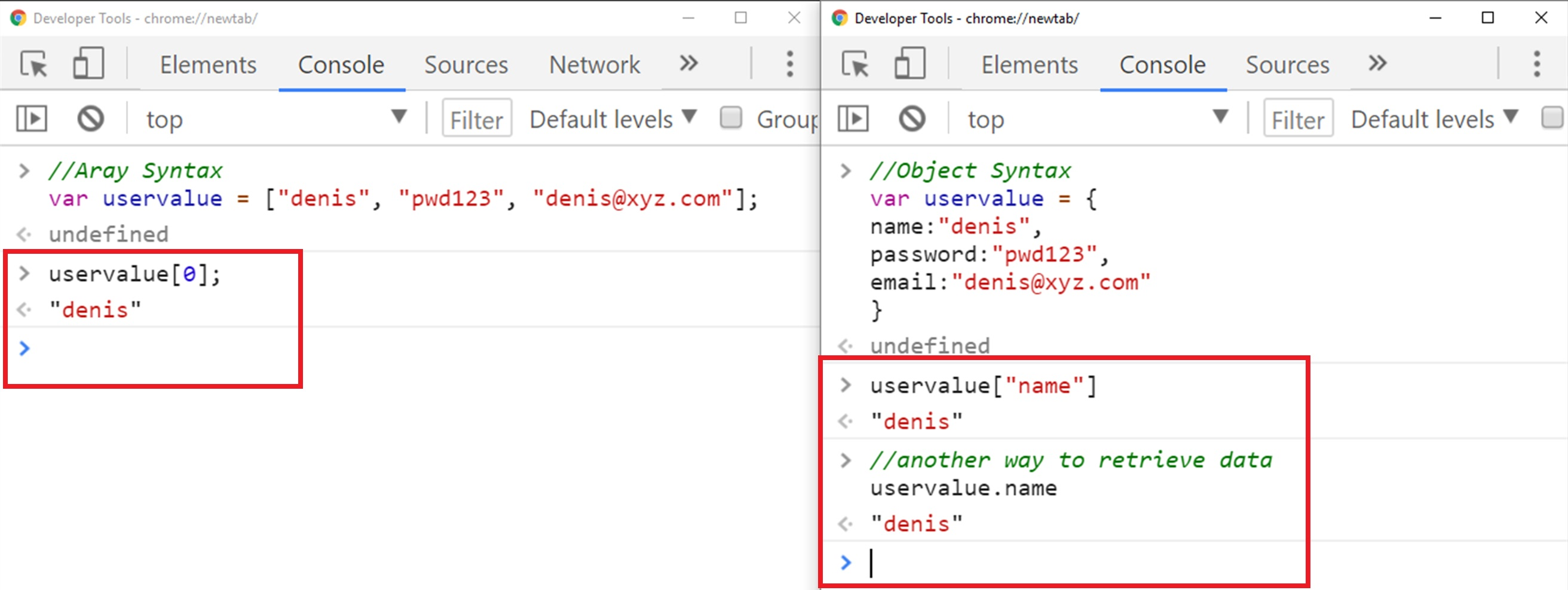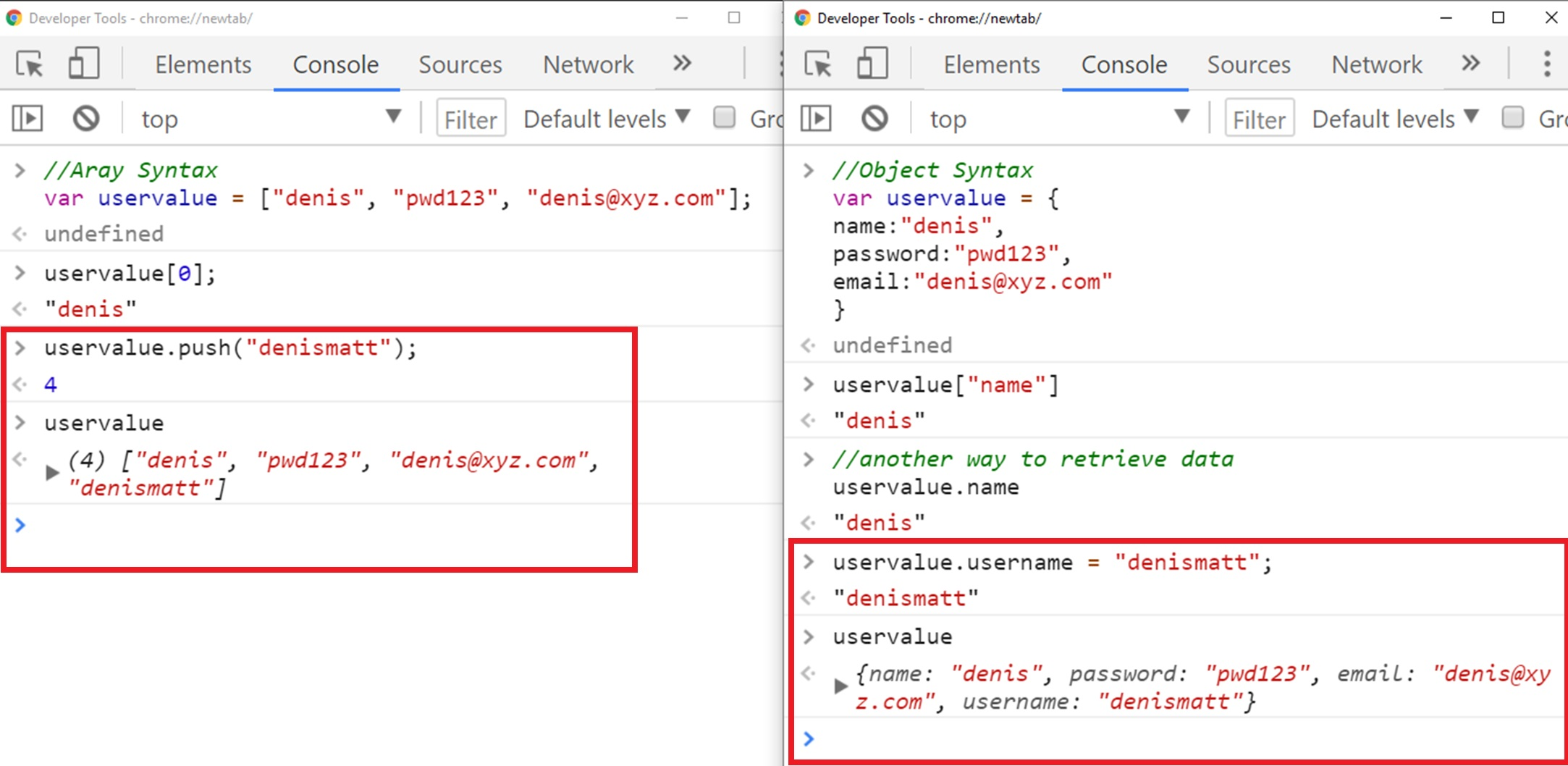# Lesson 21 Difference in Arrays And Objects in Javascript

In this lesson we are going to compare arrays and objects in JavaScript, we are going to discuss how arrays are different from the object.
We will discuss the syntax of both data structure and we will learn how we can add, retrieve data from object and array.

## The difference in Arrays and Object

So we are beginning our lesson by comparing arrays and object conceptually, keep in mind arrays is used to store a list of data and the things that we add into the array are very specific and have a particular order.
Every item is bound to an index, like the first item is bound to 0 next is with 1 and so on
On the other hand, an object is not a list, there is no particular order so object is like bunch of things floating around.
In object, we have key value pairs and key value pairs is just like dictionary, so in dictionary we have words and corresponding definition.
Just like dictionary we have keys in object which gives us corresponding value.

## Compare the Syntax of Array and Object

Now let’s compare the syntax of these two data structure type, so let’s start with the array syntax, so here I am creating an array with variable name uservalue and store name, password, and email of that user.

``````//Array Syntax
var uservalue = ["denis", "pwd123", "denis@xyz.com"];
``````

So here we make an array with three items in it, let’s compare that to making an object, so to make an object we will make a variable with uservalue and declare name, password, and email in it.
Here is the syntax for the object:

``````//Object Syntax
var uservalue = {
name:"denis",
email:"denis@xyz.com"
}
``````So here is the syntax a key name, password, and email without quotes and colon: and value and value can be anything string, number, array, another object, Boolean, undefined, so any possible value can go here.
How to retrieve data?
Now let’s compare how we can retrieve data, so if I want to retrieve data from array list and I want to retrieve username.
So I will write array name followed by the square bracket and inside the square bracket, write the index number of the name.
If I want to retrieve data from the object so remember there are two way one is similar to array write object name followed by the square bracket and inside the square bracket, write the key value name, and another way is to write object name.
which is uservalue in our case and followed by (.) dot key value uservalue.nameSo arrays are a special type of an object, where keys are always numbers, in our scenario “denis” having key value 0, pwd123 have key value 1, and denis@xyz.com have key value 2.
on the other hand in the object we have ‘name’ as the key and “denis” as the value, password as the key and “pwd123” as the value and email as the key and denis@xyz.com as the value.

So if we want to add an item into array we use array push() method and inside push method use our value.
While in object we use object name followed by dot (.) and new key.
Here is the code below for both array and object## Conclusion

So in this lesson, we have compared array and object learn how we can add, retrieve data into object and array and compare the syntax of both data structure.